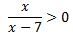# Solving Equations & Inequalities Involving Rational Functions - Quiz & Worksheet

Instructions:

question 1 of 3

### Solve. x/3 = 2

Create Your Account To Take This Quiz

As a member, you'll also get unlimited access to over 84,000 lessons in math, English, science, history, and more. Plus, get practice tests, quizzes, and personalized coaching to help you succeed.

Try it risk-free for 30 days. Cancel anytime

### 1. What are the intervals of this inequality?### 2. Which interval(s) offers a solution?Create your account to access this entire worksheet
Quizzes, practice exams & worksheets
Certificate of Completion
Create an account to get started

This quiz and attached worksheet will help gauge your understanding of equations and inequalities with rational functions. Topics you will need to know in order to pass the quiz include intervals and identifying inequalities.

## Quiz & Worksheet Goals

Use these assessment tools to measure your knowledge of:

• Solving given equations
• Defining intervals of a particular inequality
• Finding a solution for given intervals
• Define rational function
• Identify steps for rational functions

## Skills Practiced

This worksheet and quiz let you practice the following skills:

• Critical thinking - apply relevant concepts to examine information about rational functions in a different light
• Problem solving - use acquired knowledge to solve inequalities
• Interpreting information - verify that you can read information regarding equations and inequalities with rational functions and interpret it correctly

To learn more about equations and inequalities with rational functions, review the lesson Solving Equations & Inequalities Involving Rational Functions which covers the following objectives:

• Solving a rational equation
• Determining a rational inequality
Final ExamPrecalculus: High School
Status: Not Started
Chapter ExamRational Functions & Difference Quotients
Status: Not Started

Support